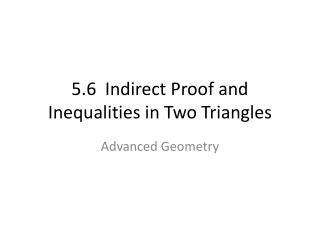# 5.6 Indirect Proof and Inequalities in Two Triangles - PowerPoint PPT PresentationDownload Presentation5.6 Indirect Proof and Inequalities in Two Triangles

5.6 Indirect Proof and Inequalities in Two Triangles
Download Presentation## 5.6 Indirect Proof and Inequalities in Two Triangles

- - - - - - - - - - - - - - - - - - - - - - - - - - - E N D - - - - - - - - - - - - - - - - - - - - - - - - - - -
##### Presentation Transcript

1. 5.6 Indirect Proof andInequalities in Two Triangles Advanced Geometry

2. HINGE THEOREM If two sides of one triangle are congruent to two sides of another triangle, and the included angle of the first is larger than the included angle of the second, then the third side of the first is longer than the third side of the second.

3. HINGE THEOREM

4. CONVERSE OF THE HINGE THEOREM If two sides of one triangle are congruent to two sides of another triangle, and the third side of the first is longer than the third side of the second, then the included angle of the first is larger than the included angle of the second.

5. Example • In triangle ABC and triangle DEF, AC = DF, BC = EF, AB = 11, ED = 15, and angle F = 58. Which of the following is a possible measure for angle C: 45, 58, 80, or 90? • In triangle GHI and triangle JKL, GH = JK, HI = KL, GI = 9, angle H = 45, and angle K = 65. Which of the following is a possible length for JL: 5, 7, 9, or 11?

6. EXAMPLE You and a friend are flying separate planes. You leave the airport and fly 200 miles due north. You then change direction and fly W 60 degrees N for 90 miles. (northwest direction, 60 degrees north of due west.) Your friend leaves the airport and flies 200 miles dues south. She then changes direction and flies E 15 degrees S for 90 miles. Each of you has flown 290 miles, but which plane is further from the airport?

7. EXAMPLE You and a friend are flying separate planes. You leave the airport and fly 120 miles due west. You then change direction and fly W 30 degrees N for 70 miles. Your friend leaves the airport and flies 120 miles due east. She then changes direction and flies E 40 degrees S for 70 miles. Each of you has flown 190 miles, but which plane is farther form the airport?

8. INDIRECT PROOF An indirect proof is a proof in which you prove that a statement is true by first assuming that its opposite is true.

9. Guidelines for Writing an Indirect Proof • Identify the statement that you want to prove is true. • Begin by assuming the statement is false; assume its opposite is true. • Obtain statements that logically follow from your assumption. • If you obtain a contradiction, then the original statement must be true.

10. Example Given: Triangle ABC Prove: Triangle ABC does not have more than one obtuse angle.

11. Example Given: Triangle ABC Prove: Triangle ABC does not have more than one right angle.